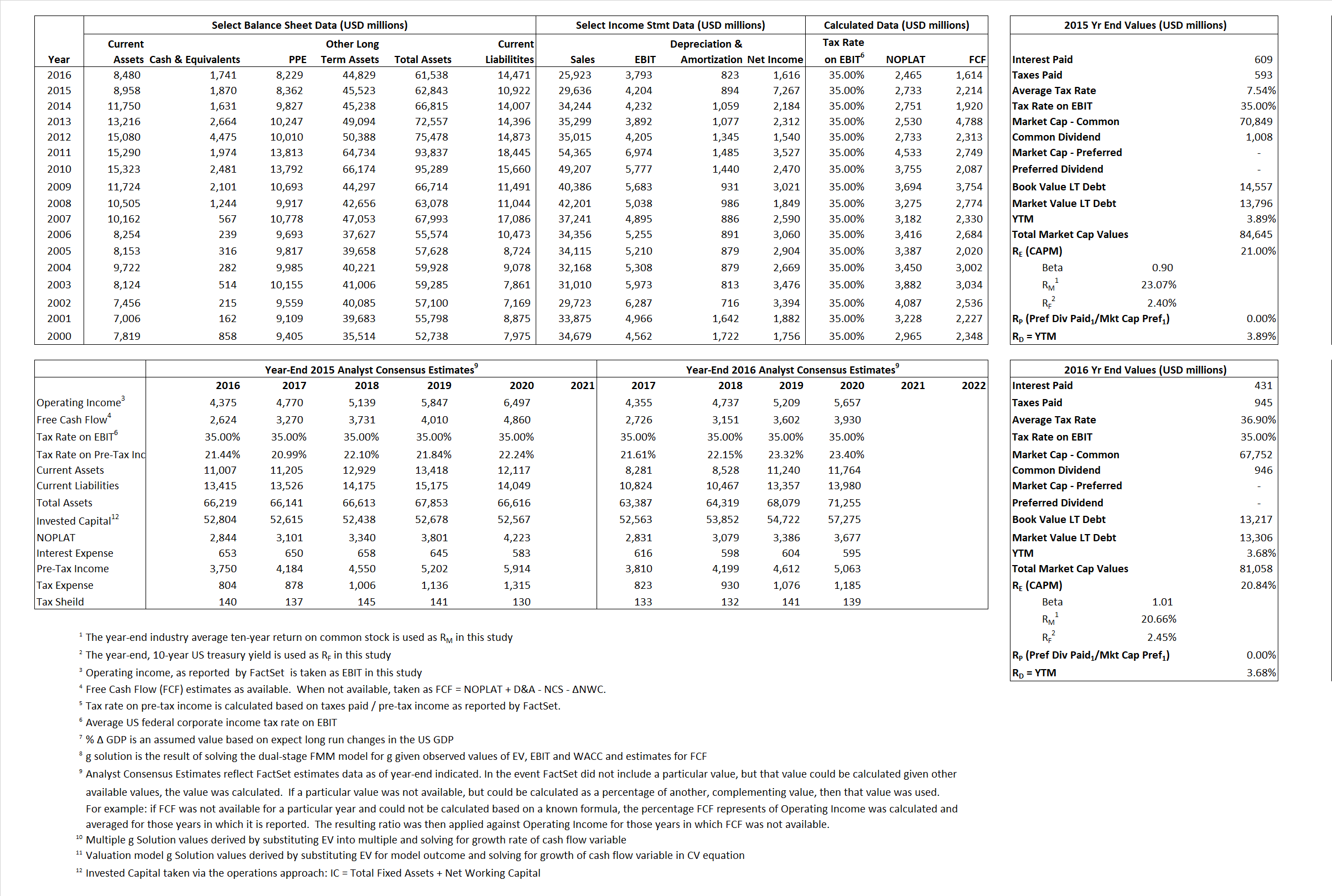# Mondolez

## Analyst Listing

The following analysts provide coverage for the subject firm as of May 2016:

 Broker Analyst Analyst Email Bernstein Research Alexia Howard alexia.howard@bernstein.com Stifel Nicolaus Christopher R. Growe growec@stifel.com RBC Capital Markets David Palmer david.palmer@rbccm.com Deutsche Bank Research Eric Katzman eric.katzman@db.com Buckingham Research Eric Larson elarson@buckresearch.com Wells Fargo Securities John J. Baumgartner john.baumgartner@wellsfargo.com Athlos Research Jonathan P. Feeney jonathan@athlosresearch.com First Global Stockbroking Kavita Thomas kavita.thomas@fglobal.com BMO Capital Markets Kenneth B. Zaslow kenneth.zaslow@bmo.com Susquehanna Financial Group Pablo Zuanic pablo.zuanic@sig.com Credit Suisse Robert Moskow robert.moskow@credit-suisse.com

## Primary Input Data## Derived Input Data

### Equational Form

Net Operating Profit Less Adjusted Taxes NOPLAT 2,733 2,465$NOPLAT\, =\, EBIT\, x\, (1 \,-\, Avg \,\,Tax\,\, Rate\,\, on\,\, EBIT)$
Free Cash Flow FCF 2,214  1,614$FCF\,=NOPLAT\,+\,Non-Cash\,Expenses-\Delta NWC\,-\,NCS$
Tax Shield TS  46 159$TS\,=\,Interest\,\,Paid\,\,x\,\, Avg \,\,Tax\,\,Rate\,\, on\,\, Pre-Tax\,\, Income$
Invested Capital IC  51,921  47,067$IC\,=\,Fixed\,\,Operating\,\,Assets\,\,+\,\,Net\,\, Working\,\, Capital$
Return on Invested Capital ROIC  5.26%  5.24%$ROIC\,=\,\frac { NOPLAT }{ IC }$
Net Investment NetInv  7  (4,031)$NetInv\,=\,{ {IC}_{1}}-{{IC}_{0}}+Depreciation$
Investment Rate IR 0.26%  -163.50%$IR\,=\,\frac {NetInv}{NOPLAT}$
Weighted Average Cost of Capital
WACCMarket  18.17%  17.80%$WACC\,=\,\frac { E }{ V } { R }_{ E }\,+\,\frac { P }{ V } { R }_{ P }\,+\,\frac { D }{ V } { R }_{ D }\left( 1- Avg\,\, Tax\,\,Rate\,\,on\,\,Pre-Tax\,\,Income \right)$
WACCBook  7.68%    7.76%
Enterprise value
EVMarket  82,775  79,317$EV\,=\,Market\,\,Cap\,\,Equity\,+\,\,Long\,\,Term\,\,Debt\,-\,Cash$
EVBook  83,665    79,228
Long-Run Growth
g = IR x ROIC
0.01%  -8.56% Long-run growth rates of the income variable are used in the Continuing Value portion of the valuation models.
g = %$\Delta$ GDP   2.50%   2.50%
Margin from Operations M  14.19%  14.63%$M\,\,=\,\,\frac{EBIT}{SALES}$
Depreciation/Amortization Rate D  17.54%  17.83%$D\,\,=\,\,\frac{D+A}{EBITDA}$

## Valuation Multiple Outcomes

The outcomes presented in this study are the result of original input data, derived data, and synthesized inputs.

### model g solution

12/31/2015 12/31/2016 12/31/2015 12/31/2016 12/31/2015 12/31/2016

EV/SALES$\frac {EV}{Sales} \,= \,\frac{ROIC\, -\, g}{ROIC\,(WACC\,-\,g)}\,(1\,-\,T)\,(M)$

2.79  3.06  39.88%  36.14% 24.07%  23.40%

EV/EBITDA$\frac {EV}{EBITDA} \,= \,\frac{ROIC\, -\, g}{ROIC\,(WACC\,-\,g)}\,(1\,-\,T)\,(1\,-\,D)$

16.24 17.18 39.88%  36.14% 24.07% 23.40%

EV/NOPLAT$\frac {EV}{NOPLAT} \,= \,\frac{ROIC\, -\, g}{ROIC\,(WACC\,-\,g)}$

30.29 32.17 39.88% 36.14% 24.07% 23.40%

EV/FCFOPS$\frac {EV}{FCF_{OPS}} \,= \,\frac{ROIC\, -\, g}{ROIC\,(WACC\,-\,g)}\,(1\,-\,T)$

37.39 49.14 39.88% 36.14% 24.07% 23.40%

EV/EBIT$\frac {EV}{EBIT} \,= \,\frac{ROIC\, -\, g}{ROIC\,(WACC\,-\,g)}\,(1\,-\,T)$

19.69 20.91 39.88% 36.14% 24.07% 23.40%

EV/IC$\frac {EV}{IC} \,= \,\frac{ROIC\, -\, g}{WACC\,-\,g}$

1.59 1.69 39.88% 36.14% 24.07% 23.40%